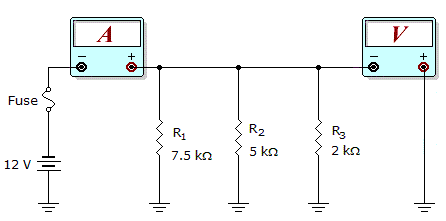# Electronics - Parallel Circuits - Discussion

Discussion Forum : Parallel Circuits - General Questions (Q.No. 11)
11.What would these meter readings indicate about the circuit in the given circuit?

Meter Readings: I = 7.6 mA, V = 12 V

R1 is open.
R2 is open.
The fuse is open.
The circuit is operating normally.
Explanation:
No answer description is available. Let's discuss.
Discussion:
17 comments Page 1 of 2.

Anomie said:   4 years ago
I=7.6mA so.
~7.6=12V/(7.5K*2K/9.5K).
So R2 is not considered for the meter current so it can a open R2.
(1)

Muhammad Feroz Aftab said:   5 years ago

Saish said:   6 years ago
Yes, you are correct. Agree @Addy.
(1)

Maan said:   6 years ago
I agree @Saichaitanya.

Pratiksha said:   7 years ago
Well said, thanks for the given explanation.

Just look at the fig. above, there is showing batteries positive lead is connected to ground. Not a short to another component which is also grounded. If positive supply connect to the ground else is there current flow in the circuit! Please tell me.

Samir said:   7 years ago
Thank you guys for giving a clear explanation.

Pre said:   7 years ago
Well done @ Himanshu Verma.

Santosh said:   9 years ago
Voltmeter is in parallel connection, so reading is 12V.

Now for resistance calculation which is connected parallel with 2k ohm resistance (R3):

Equivalent resis. R = 12V/7.6mA = (30/19)K ohm.
So, Rx ll R3 = (30/19)k.
or, (1/Rx)+ (1+R3) = 1/(30/19)k.
or, (1/Rx)+ (1/2) = (19/30).
or, (1/Rx) = (19/30)-(1/2).
or, 1/Rx = 4/30.
or, Rx = 30/4 = 7.5k ohm.

i.e., shows R1 is connected & R2 is opened.